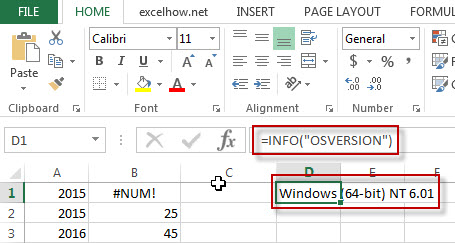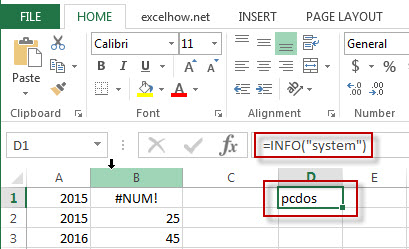# Excel Info Function

This post will guide you how to use Excel INFO function with syntax and examples in Microsoft excel.

### Description

The Excel INFO function returns information about the operating environment.

The INFO function is a build-in function in Microsoft Excel and it is categorized as an Information Function.

The INFO function is available in Excel 2016, Excel 2013, Excel 2010, Excel 2007, Excel 2003, Excel XP, Excel 2000, Excel 2011 for Mac.

### Syntax

The syntax of the INFO function is as below:

= INFO (type_text)

Where the INFO function arguments are:
Type_text -This is a required argument. The type of information that you want to return about the operating environment.

The following type values can be used:

 Type_text Returns “directory” Path of the current directory or folder. “numfile” Number of active worksheets in the open workbooks. “origin” Returns the absolute cell reference of the top and leftmost cell visible in the window, based on the current scrolling position, as text prepended with “\$A:”. This value is intended for for Lotus 1-2-3 release 3.x compatibility. The actual value returned depends on the current reference style setting. Using D9 as an example, the return value would be: ·         A1 reference style     “\$A:\$D\$9”. ·         R1C1 reference style    “\$A:R9C4” “osversion” Current operating system version, as text. “recalc” Current recalculation mode; returns “Automatic” or “Manual”. “release” Version of Microsoft Excel, as text. “system” Name of the operating environment: Macintosh = “mac” Windows = “pcdos”

From Microsoft website

### Example

The below examples will show you how to use Excel INFO Function to return the information about the operating environment.

#1 =INFO(“OSVERSION”)Note: the above excel formula will return the current operating system version.

#2 =INFO(“system”)Note: The above excel formula will return the name of the current operating environment.

Related Posts

Excel TYPE Function

This post will guide you how to use Excel TYPE function with syntax and examples in Microsoft excel. Description The Excel TYPE function returns the type of a value. So you can use the TYPE function to return an integer ...

Excel N Function

This post will guide you how to use Excel N function with syntax and examples in Microsoft excel. Description The Excel N function converts a value to a numeric value. And the N function can be used to convert the ...

Excel ISREF Function

This post will guide you how to use Excel ISREF function with syntax and examples in Microsoft excel. Description The Excel ISREF function used to check if a value is a valid reference. If so, returns TRUE; if the value ...

Excel ISNONTEXT Function

This post will guide you how to use Excel ISNONTEXT function with syntax and examples in Microsoft excel. Description The Excel ISNONTEXT function used to check if a value is text. If so, returns FALSE; if the text is not ...

Excel ISTEXT Function

This post will guide you how to use Excel ISTEXT function with syntax and examples in Microsoft excel. Description The Excel ISTEXT function used to check if a value is text. If so, returns TRUE; if the text is not ...

Excel ISNA Function

This post will guide you how to use Excel ISNA function with syntax and examples in Microsoft excel. Description The Excel ISNA function used to check if a cell contains the #N/A error, if so, returns TRUE; otherwise, the ISNA ...

Excel ISERR Function

This post will guide you how to use Excel ISERR function with syntax and examples in Microsoft excel. Description The Excel ISERR function returns TRUE if the value is any error value except #N/A. The ISERR function is a build-in ...

ISNUMBER Function in Excel

This post will guide you how to use ISNUMBER function with syntax and examples in Microsoft excel. Description The Excel ISNUMBER function returns TRUE if the value in a cell is a numeric value, otherwise it will return FALSE. so you can ...

Excel ISError Function

This post will guide you how to use Excel ISERROR function with syntax and examples in Microsoft excel. Description The Excel ISERROR function used to check for any error type that excel generates and it returns TRUE for any error ...

Excel ISBlank Function

This post will guide you how to use Excel ISBLANK function with syntax and examples in Microsoft excel. Description The Excel ISBLANK function returns TRUE if the value is blank or null. The ISBLANK function is a build-in function in Microsoft ...

Sidebar